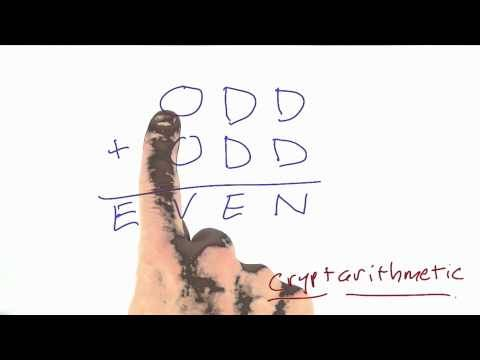Can you illustrate the concept of digimetic and skeletal division cryptarithms with a solved example for each?

Since they are both forms of cryptarithm, I should probably start by defining that.

What is a cryptarthim?In the most simplest terms, a cryptarthim is a verbal-based mathematical problem. The figures in the mathematical problem are thus replaces by letters of the alphabet.

Digimetic problems generally a cryptarithm in which digits are used to replace other digits.

Skeletal division is a "long division" where almost all the digits are replaced by symbols (most commonly asterisks).

I may need someone to jump in with some solved examples for you though, because I don't think I'll be solving any cryptarithms any time soon!

thanked the writer.